OF AN ITERATIVE PROCEDURE FOR SOLVING THE OPTIMIZATION PROBLEM INTO AN ELLIPSOID

# Liljana Stefanovska

Faculty of Technology and Metallurgy, P.O. Box 580, Skopje, Macedonia

liljana@ereb1.mf.ukim.edu.mk

# Beti Andonovic

Faculty of Technology and Metallurgy, P.O. Box 580, Skopje, Macedonia

band@freemail.com.mk

## Sonja Gegovska-Zajkova

Faculty of Electrical Engineering, P.O. Box 574, Skopje, Macedonia

szajkova@cerera.etf.ukim.edu.mk

ABSTRACT. For solving the problem of optimization in a space limited by an ellipsoid and a linear goal function, for which the maximum is searched, there is an iterative procedure suggested. The procedure starts from an interior point of the ellipsoid and by moving into its interior there is a generated sequence of points of the contour of the ellipsoid that converges to the optimal solution (point), where the goal function is of greatest value.

#### 1. PRELIMINARY OBSERVATION

Let Rn is Euclidean n-dimensional space where the vector xÎRn is denoted by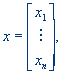where xi (i = 1, ..., n) are scalars.

The inner product of two vectors x, y Î Rn is defined byand the norm of an arbitrary vector xÎRn  is defined by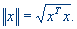For any vector x¹0, there is its unit vectorthat can be determined by the relationwhere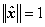.

2. SETTING OF THE OPTIMIZATION PROBLEM

We observe the optimization problem of the following type

max{cTx | xÎE}

E = {xÎRn | xTAx £ 1}                                                 (1)

where c, xÎRn  are vectors, A is a diagonal matrix of positive elements of an order n´n, that iswhere ai (i = 1, ..., n)  are scalars. Such a defined feasible space determines the interior of an n-dimensional ellipsoid.

Not getting more specific, instead of problem (1), we observe the problem of the following type

max{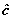Tx | xÎE}

E = {xÎRn | -xTAx ³ -1}                                            (2)

whereis the unit vector and the inequality -xTAx ³ -1 enables the normal vectors of the tangent plane to ellipsoid to be oriented to the interior of the ellipsoid (feasible space). The valuesof the goal function of the problem (2) and z = cTx of the goal function of the problem (1) are connected by the following relation3. AN ALGORITHM OF THE METHOD

To solve the optimization problem (2), there is an iterative procedure suggested for constructing a sequence of feasible solutions {x(k)} obtained by the recurrence formula

x(k+1) = x(k)  + t(k) d(k),    ( k = 0, 1, 2, ... ).                                 (3)

The vector d(k)¹0 determines the feasible movement direction into the interior of the ellipsoid, and the scalar t(k)¹0 determines the length of the step which is chosen such that the following solution is on the contour of the feasible area and thereat a real improvement in the goal function is achieved. That means that the following inequality is satisfiedTx(k+1) >Tx(k).                                                                      (4)

3.1. THE STARTING STEP

The method starts from an interior feasible solution x(0), i.e.

- x (0)TAx(0) > -1.

A starting movement direction is the vector

d(0) =,                                                                                   (5)

and the length of the step t(0) is chosen by the condition that the next solution x(1) obtained by

x(1) = x(0) + t(0) d(0)                                                                    (6)

satisfies the following equality

x(1) TAx(1) = 1,

i.e., to be a boundary feasible solution. The graphic review of this coming out on the contour of the feasible space in R2 is shown on Figure 1.Figure 1.

Indeed, by choosing the starting movement direction (5), there is always some small length of the step t(0) > 0, provided by the condition  x(0) to be an interior solution, where for the next solution

x(1) = x(0) + t(0)the value of the goal function will be(1) =Tx(1) =T  (x(0) + t(0)) =Tx(0) + t(0)T=Tx(0) + t(0)  >Tx(0) =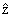(0).

Thus, for the next solution x(1) the value of the goal function will increase, which means that a better feasible solution is obtained.

3.2. AN ARBITRARY STEP

The further determining of the boundary feasible sequence of solutions

x(i+1) = x(i)  + t(i) d(i)                   (i = 1, 2, ... )                             (7)

continues by choosing an upper movement direction and determining the step length. At any feasible solution x(i) determined by (7) and for which holds

x (i)TAx(i) = 1

there is the vector n(i)ÎRn, that is perpendicular to the tangent plane of the ellipsoid at the vector x(i) and directed to the interior of the ellipsoid. Thus,andand  ||(i)|| = 1. We give the following

Lemma: The direction

d(i) =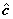+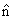(i)                                                                           (9)

is a feasible movement direction (Figure 2.), by which the goal function increases its value.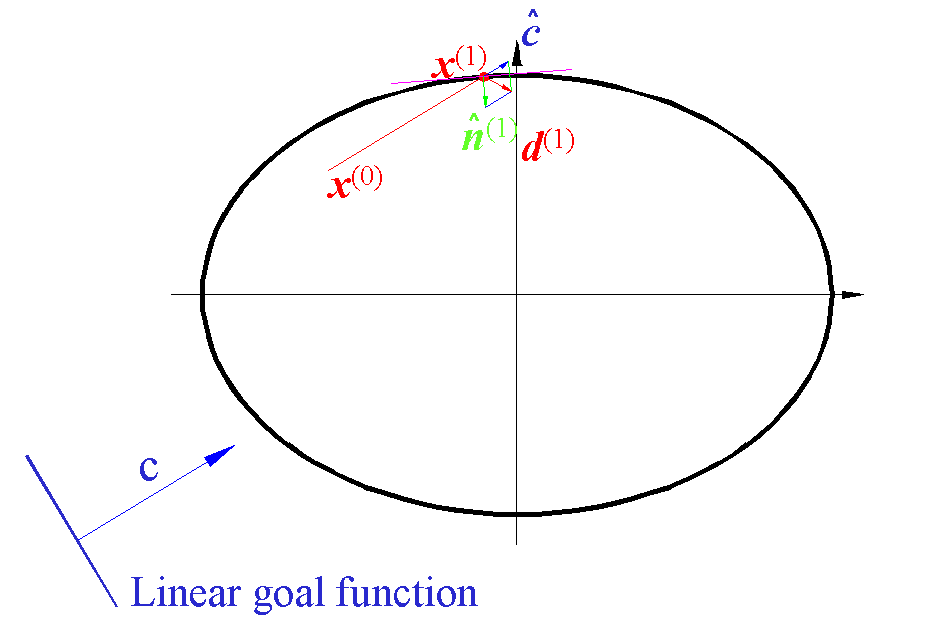Figure 2

Proof: For a length that is small enough t > 0, the next feasible solution is

x(i+1) = x(i) + t d(i) = x(i) + t (+(i))

for which it is true

x(i+1)TAx(i+1) = 1

and the value of the goal function(i+1) =Tx(i+1) =T(x(i) + t (+(i))) =

=Tx(i) + t (T+T(i)) =(i) + t (1+T(i)) >(i)

achieves a real increasement because

|||| = ||(i)|| = 1.

To the sequence of boundary solutions

there is a corresponding sequence of the goal function values

{ z(i) }, (i = 0, 1, 2,  …)

for which it is true

z(i+1) > z(i).

The method ends when the following condition is satisfied

|| z(i+1)  -  z(i) || < e .                                                       (10)

for an arbitrary e > 0.

4. EXAMPLE

In R2, let the goal function vector beand the ellipsoid is defined by the diagonal matrix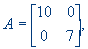where e =0.001 is given.

For the following three different initial interior solutions x(0), obtained results are presented in three different tables correspondingly:

Table 1.  Results for the initial solutioni = 0 i = 1 i = 2 i = 3 i = 4 x(i)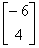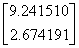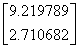d(i)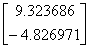z(i) - 18 15.54749 53.06996 54.230130 54.230990

Table 2. Results for the initial solutioni = 0 i = 1 i = 2 i = 3 i = 4 x(i)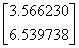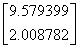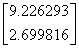d(i)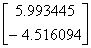z(i) - 48 37.450370 53.923340 54.230910 54.230990

Table 3. Results for the initial solutioni = 0

i = 1

i = 2

i = 3

i = 4

x(i)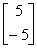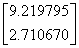d(i)z(i)

10

40.062430

54.229780

54.230980

54.230620

The results presented above show that the optimal solution is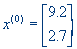for which the goal function reaches the maximum in the fourth iterative step (i = 4), and zmax = 54.23.

REFERENCES

  Bandi, B.,  Metodì optimizacii, Vvodnìi kurs, Izd. Radio i svyazy, Moskva, 1988

  Chang, Y. S., The Steepest Descent Gravitational Method for Linear Programming, Discrete Applied Mathematics 25, (1989) 211-239

  Garvin, W. W., Introduction to Linear Programming, Mc Graw-Hill Book Company, Inc, 1960

 Karcicka, L. D., Teorija i metodi na linearnoto programirane, Univerzitet Kiril i Metodij, Skopje, 1987

  Zlobec, S., Petric, J., Nelinearno programiranje, Naucna knjiga, Beograd, 1989

  Vujcic, V., Asic;, M., Milicic, N., Matematicko programiranje, Matematicki institut, Beograd, Savremena racunska tehnika i njena primena, Knjiga 7, 1980